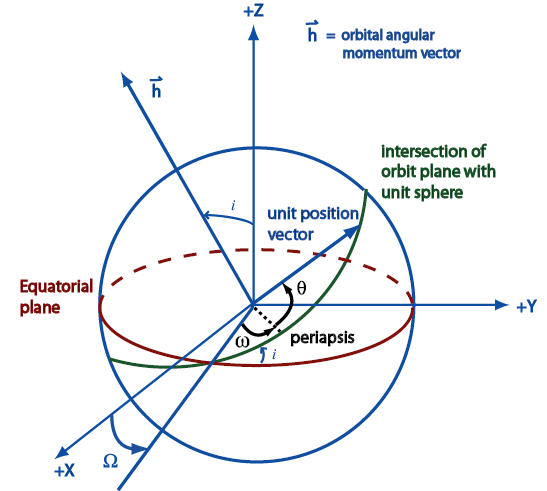Gets the right ascension of the ascending node of the orbit, in radians.

Namespace:  AGI.Foundation.Coordinates
Assembly:  AGI.Foundation.Core (in AGI.Foundation.Core.dll) Version: 20.2.404.0 (20.2.404.0)Syntax
`public double RightAscensionOfAscendingNode { get; }`

#### Property Value

Type: DoubleRemarks

The ascending node is the point where the satellite crosses the equator moving south to north. In the diagram below, this point is referenced from the +X direction, which points in the Vernal Equinox direction. The angle between the X axis and the ascending node is called the right ascension of the ascending node (RAAN), represented here by Ω.In the above diagram, ω is the argument of periapsis, θ is the true anomaly, and i is the inclination.See Also